# 【优化求解】基于遗传算法优化PARSEC 方法的翼型形状附matlab代码

+关注继续查看

## 2 仿真代码

%initial individual

close all

clc

clear all

% p0=[0.0155 0.296632 0.060015 -0.4515 0.296632 -0.06055 0.453 0 0.001260 0 7.36]; %%NACA 0012

p0=[0.0216 0.3445 0.07912 -0.6448 0.17 -0.033797 0.6748 0 0 0 0];  %%NACA 2411

range=[0.0015 0.025 0.015 -0.01 0.02 -0.015 0.075 0 0 -0.175 0.05];

%range=[0.02 0.023 0.32 0.37 0.077 0.08 -0.63 -0.65 0.15 0.19 -0.02 -0.05 0.6 0.75 0 0 0 0 -4.55 -4.9 15 15.1];

%Solver parameters

Npanel=200;

uinf=1;

AOA=5*pi/180;

genNo=20;       %number of generations

%Genetic solution

[cloriginal,clfittest,fittest]=GAairfoil(genNo,p0,range,uinf,AOA,Npanel);

%ploting and graphing

fprintf(' Original   Cl= %f \n Optimized  Cl= %f \n',cloriginal,clfittest)

figure

graphCl(fittest,Npanel,uinf,5,'k');

graphCl(p0,Npanel,uinf,5,'r');

legend('Optimized','original')

xlabel('AOA')

ylabel('Cl')

line([-100 100],[0,0],'color','k','LineWidth',1)

line([0,0],[-10 10],'color','k','LineWidth',1)

title('Coeffecient of lift vs. Angle of attack')

grid on

## 3 运行结果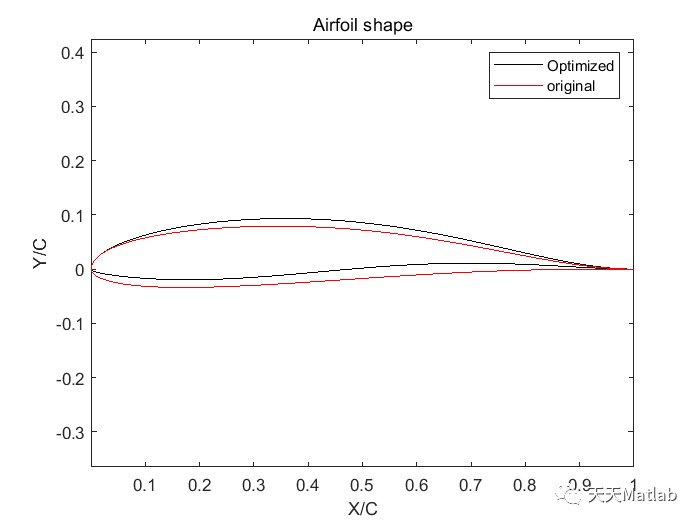编辑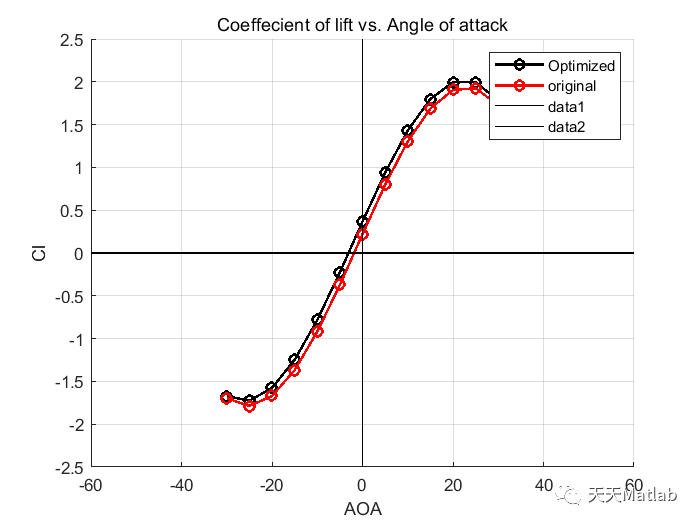编辑

## 4 参考文献

方静. 基于遗传算法的翼型优化[D]. 哈尔滨工程大学, 2007.

### 博主简介：擅长智能优化算法、神经网络预测、信号处理、元胞自动机、图像处理、路径规划、无人机等多种领域的Matlab仿真，相关matlab代码问题可私信交流。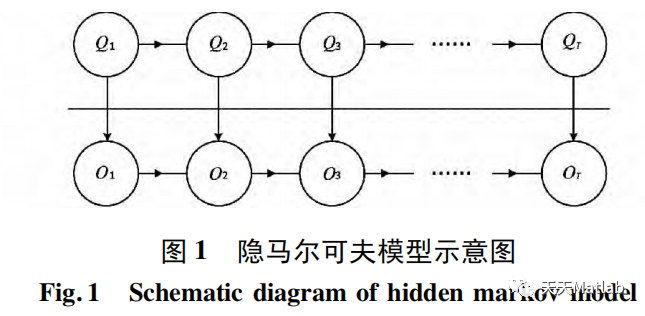【图像去噪】基于隐马尔可夫模型实现图像去噪处理附matlab代码
【图像去噪】基于隐马尔可夫模型实现图像去噪处理附matlab代码
30 0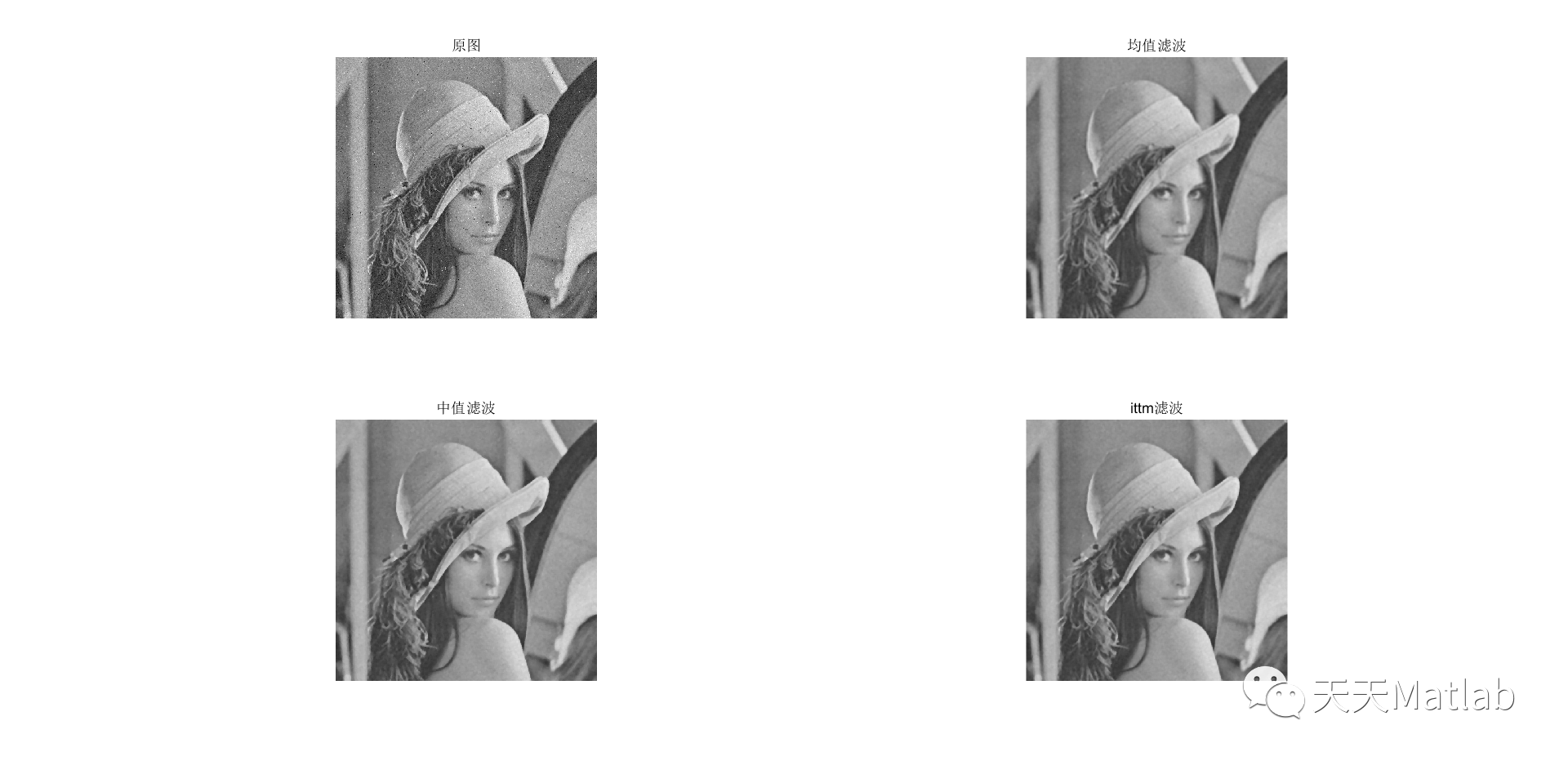【图像去噪】基于ITTM 滤波器实现图像去噪附matlab代码
【图像去噪】基于ITTM 滤波器实现图像去噪附matlab代码
24 0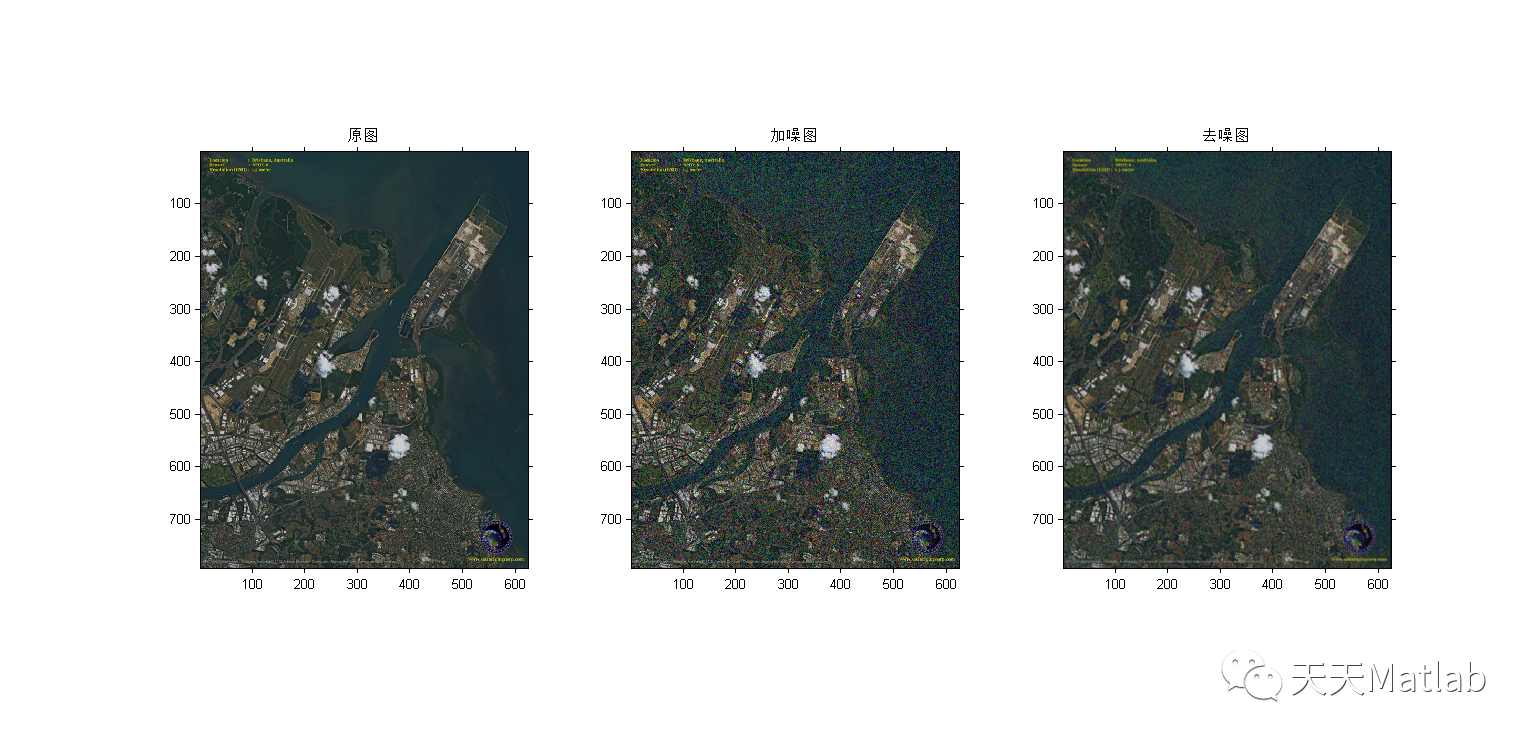【图像去噪】基于自适应布谷鸟算法优化维纳滤波器实现多光谱图像去噪附matlab代码
【图像去噪】基于自适应布谷鸟算法优化维纳滤波器实现多光谱图像去噪附matlab代码
61 0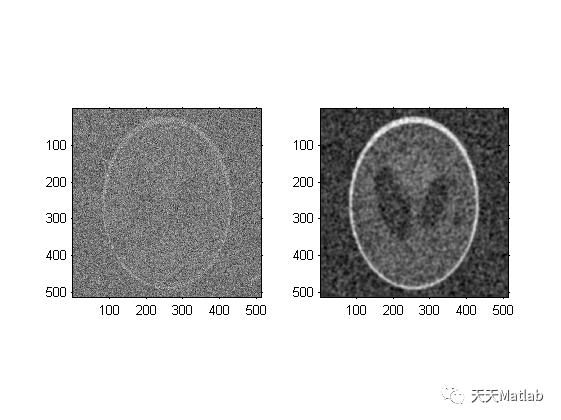【图像去噪】基于各向异性滤波实现图像去噪附matlab代码
【图像去噪】基于各向异性滤波实现图像去噪附matlab代码
19 0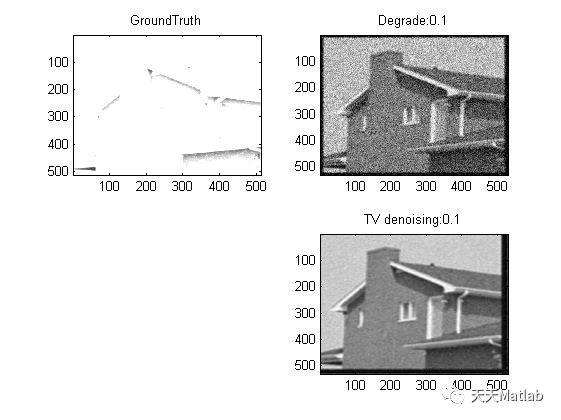【图像去噪】基于正则化实现图像去噪附matlab代码
【图像去噪】基于正则化实现图像去噪附matlab代码
34 0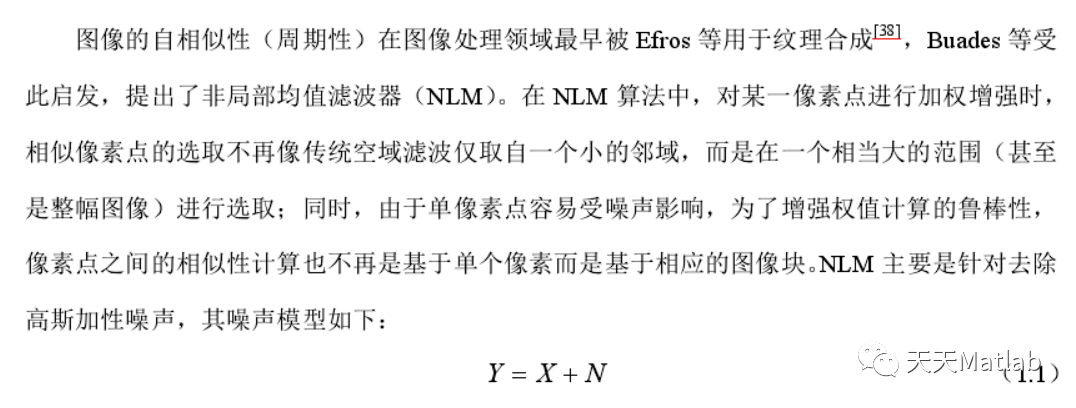【图像去噪】基于非局部欧几里德中值 (NLEM) 实现图像去噪附matlab代码
【图像去噪】基于非局部欧几里德中值 (NLEM) 实现图像去噪附matlab代码
14 0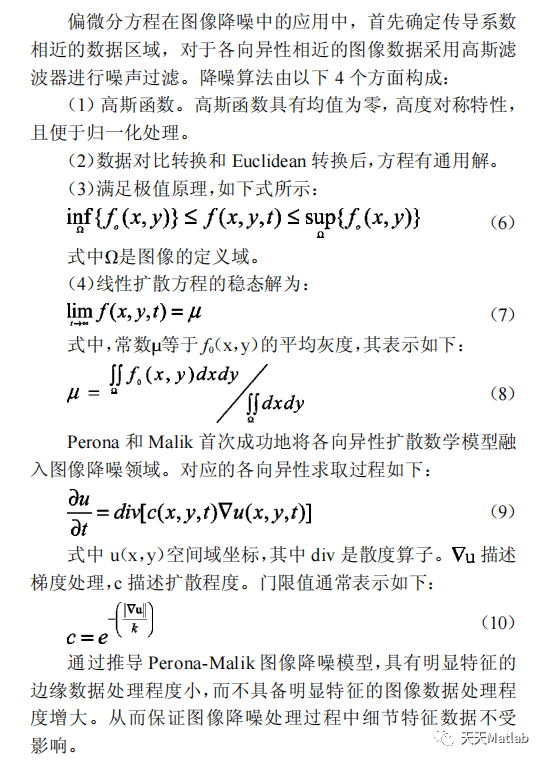【图像去噪】基于偏微分方程(PDE)实现图像去噪附matlab代码
【图像去噪】基于偏微分方程(PDE)实现图像去噪附matlab代码
34 0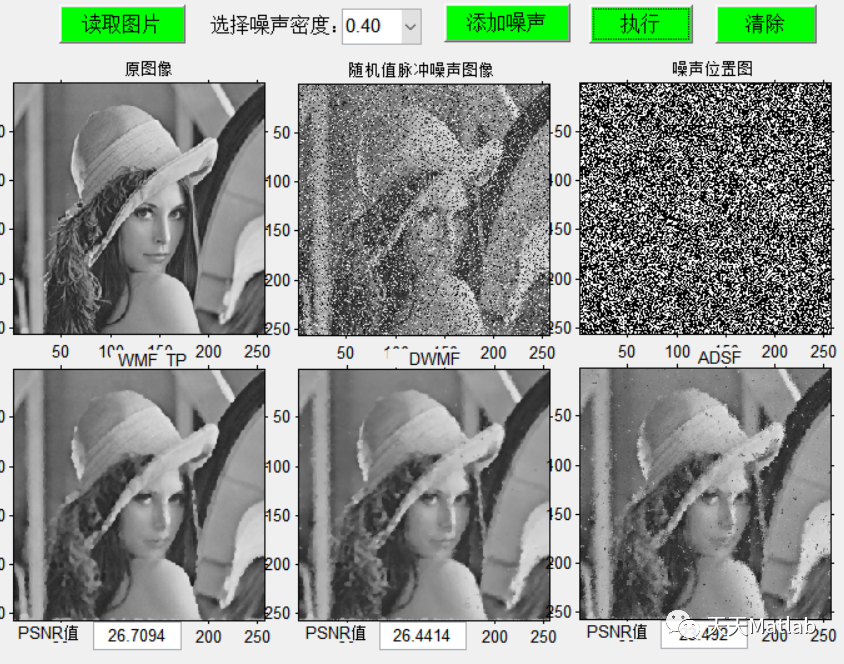【图像去噪】基于绝对差分中值滤波、加权中值滤波法、改进加权中值滤波实现脉冲噪声图像去噪附matlab代码
【图像去噪】基于绝对差分中值滤波、加权中值滤波法、改进加权中值滤波实现脉冲噪声图像去噪附matlab代码
30 0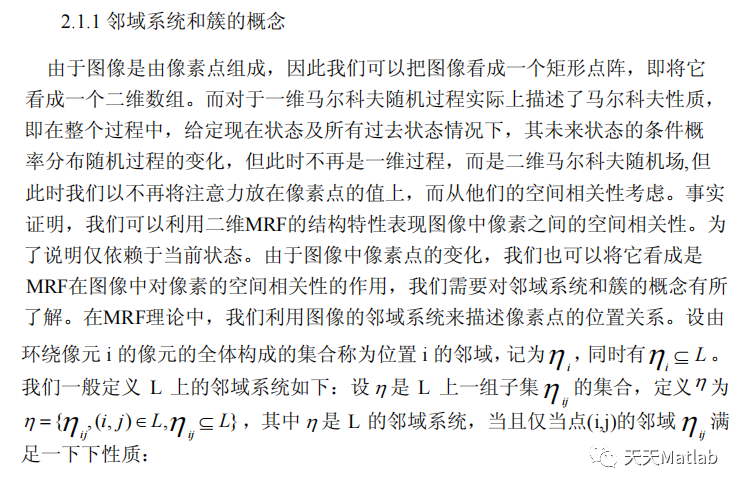【图像去噪】基于马尔可夫随机场实现图像去噪附matlab代码
【图像去噪】基于马尔可夫随机场实现图像去噪附matlab代码
56 0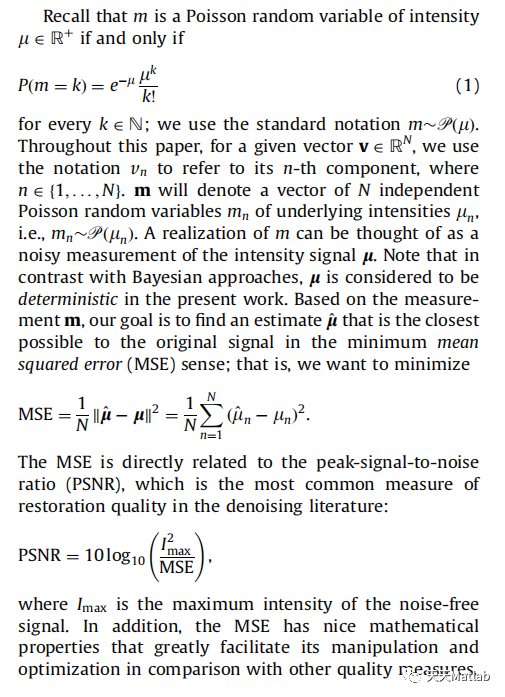【图像去噪】基于快速跨尺度小波降噪实现泊松损坏图像去噪处理附matlab代码
【图像去噪】基于快速跨尺度小波降噪实现泊松损坏图像去噪处理附matlab代码
12 0+关注
Matlab科研工作室

343

0